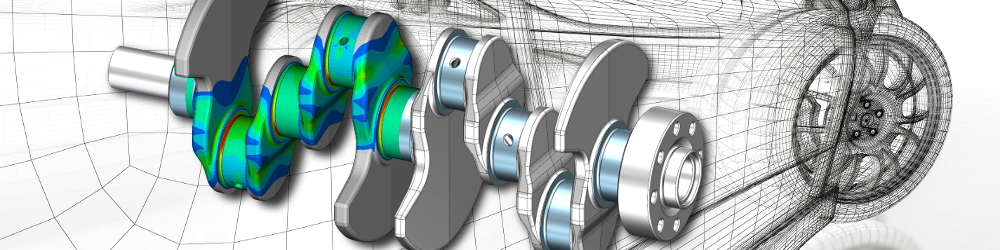•• 1

# Static structural analysis

In the field of mechanics, statics deals with the resting system. A change in forces over time is not present or is negligible from a technical point of view. Thus, the forces and their effects in a state of equilibrium are examined. Statics is therefore a special case of dynamics. Due to the lack of time dependence, the general equation of motion is reduced to the displacement component. The terms for inertial forces and speed-dependent damping are omitted.

If only certain states are of interest for the technical evaluation of a structure, dynamically loaded systems can be transferred to a static view. Such a case is called a quasistatic calculation.

If non-linearities are excluded from the consideration, one speaks of a linear static FEM calculation, the simplest conceivable application case. The loads can be applied in one step and the system of equations can be solved directly. If the model is formally sufficiently supported, there is always a solution.

However, if non-linear effects, such as plasticity or contact, have to be taken into account in the analysis, the load is usually applied in small steps. The final solution is approximated iteratively. Depending on the model quality, the degree of non-linearity and the selected step size, divergence and thus premature termination of the calculation is possible.

## Computation time / model size

Compared to transient analysis, the omission of the two time-dependent terms in the general equation of motion leads to significantly shorter computing times and the hardware requirements are significantly lower. This advantage can be used to examine more variants of a system in the same development time, or the system under consideration with a higher degree of detail.

## Relevance

Static and quasistatic, linear and nonlinear FEM structure analyses are the most frequently used type of calculation in many application areas.Warmesberg 24, 9450 Altstätten, Switzerland

+41 79 471 1612